# EIGRP Load Balancing for IPv4

EIGRP is a Cisco proprietary protocol for routing. Router enabled EIGRP calculates the best route to a destination using the metric value that is based on the link bandwidth and delay. The protocol selects the shortest path and installed that path in the routing table. If the destination has more than one path with the same administrative distance and a metric value; all least-metric and same routes are installed in the routing table.

When there are several routes with equal metric value, by default all dynamic routing protocols, including EIGRP, forward packets using load balancing.  We can configure EIGRP to install multiple paths even if the metric is not equal. This is the only protocol which supports unequal cost load balancing. There are two types of load balancing:

When a router has two or more paths to a destination with equal cost metrics, then the router forwards the packets using all paths equally called equal-cost load balancing. The protocol should have an equal administrative distance to a destination when forwarding packets. Using load balancing, the router uses network segments and bandwidth more efficiently.

By default, Cisco IOS Software can balance the load across up to four equal-cost paths. We can configure the EIGRP load balancing up to 16 equal-cost paths using the “maximum-path” in the router configuration mode. The router can keep up to 32 equal-cost routes in the routing table. If we set the maximum-path value to 1, this means that the equal-path load balancing is disabled on the router. The Cisco router uses two types for balancing the network load, per-destination basis, and per-packet basis.   The command syntax for equal-cost EIGRP load balancing configuration is:

Router(config-router)# maximum-paths value

We can verify the EIGRP load balancing using the “show ip protocols command. The command displays the number of equal-cost paths currently configured on the router. Cisco IOS, by default, allows EIGRP load balancing for four equal-cost paths routes. The output in Figure 1 illustrates the topology for that we will use in this lesson and figure 2 illustrate that R1 is using the default equal-cost EIGRP load balancing.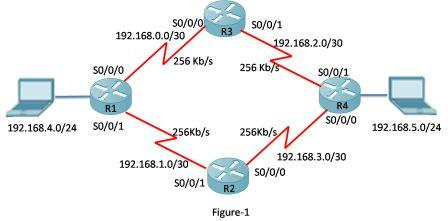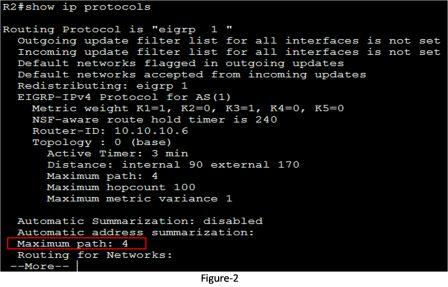Figure 3 illustrates the routing table of R1. The Router R1 has two successors for network 192.168.5.0/24 via 192.168.0.2 and via 192.168.1.2. The metric for both routes are equal, so both are installed in the routing table. This is the example of equal-cost load balancing. By default, EIGRP uses four routes with equal cost in the routing table.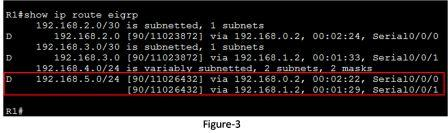If routing metrics are not equal then load balancing will not take place automatically. So a command called “variance” needs to be used for configuring a load-balancing. This command makes EIGRP load balance on unequal metrics values. The command syntax for unequal load-balancing is:

Router(config-router)variance  < Metric variance Multiplier>

The command will enable EIGRP to install multiple loop-free routes with unequal cost in a local routing table. A route learned through EIGRP should meet two criteria to be installed in the local routing table:

• The route should be loop-free, being both a feasible successor (FS) or having a reported distance (RD) that is less than the total distance.
• The metric of the route must be lower than the metric of the best route (the successor) multiplied by the variance configured on the router.

If the variance value is set to 1 on the router then only routes with the same metric are installed as the successor in the local routing table, this is called equal-cost load balancing. But if the variance is set to 2, any EIGRP- learned route with a metric less than 2 times the successor metric will also be installed in the local routing table as successor.Figure 4 illustrates that the R1 has two unequal links to reach network 192.168.5.0. The links via R3 has 256 kb/s and the link via R2 has 128 Kb/s speed. We know that the EIGRP installs the route with the lowest cost in the routing table. Let’s check the routing table of R1 in figure 5.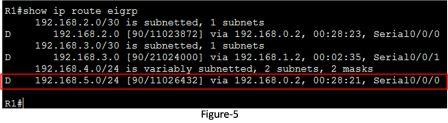Now let’s configure the R1 with variance command. We know that the link with R2 is 2 times slower than the link with R3. So the value of variance should be 2. The range of the variance that we can configure is from 1 to 128. Figure 7 illustrates the configuration of variance and the routing table of R1 after variance configuration.  You can see that two routes are installed for destination 192.168.5.0/24 network; even the metric is not equal. This is unequal load balancing.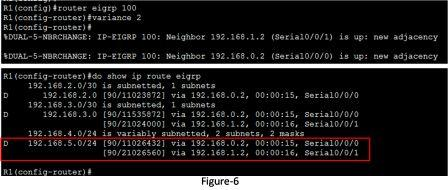We can also examine the topology table with the “show ip eigrp topology” to verify to successor routes for this destination. Figure 7 illustrates the output of this command.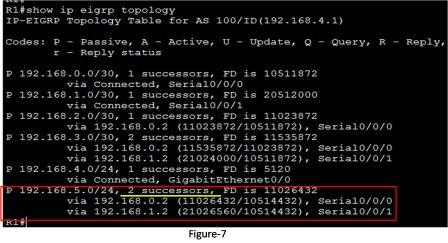We can control the traffic among routes in case multiple routes for the same destination network that has different costs. The command “trafﬁc-share balanced” command can distribute traffic proportionately to the ratio of the cost.

(Visited 66 times, 66 visits today)